GRE Subject Test: Math : Standard Deviation

Example Questions

Example Question #1 : Standard Deviation

What is the standard deviation of the numbers 3, 5, 8, 9, and 10?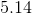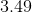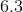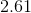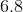Explanation:

The first step with standard deviation is to find the average or mean of the numbers. Add 3+5+8+9+10 together to get 35. Divide that by the number of scores, which is 5, to get a mean of 7.

Now, subtract the mean from each of the numbers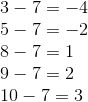Next, square those values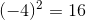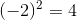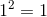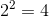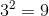Now find the mean of these squared differences by adding the values and then dividing by the amount of numbers, 5 in this case.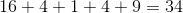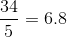Finally, take the square root of this number to get the standard deviation.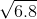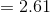All GRE Subject Test: Math Resources